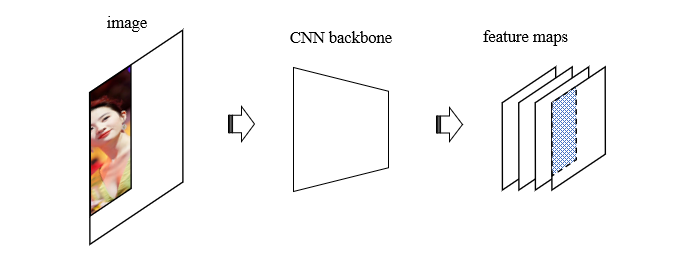Fenrier Lab

# 如何在神经网络中实现不同尺寸图片的批量输入

transform = transforms.Compose(
[
transforms.ToTensor(),
transforms.Resize(256),
transforms.RandomCrop(224),
...
]
)\left[ \begin{aligned} a &\quad b & c\\ e &\quad f \end{aligned} \right]

def preprocess(self, batched_inputs: List[torch.Tensor]):
"""
Args:
batch_inputs: 图片张量列表
Return:
image_sizes_orig: 原始图片尺寸信息
"""
## 保留原始图片尺寸
image_sizes_orig = [[image.shape[-2], image.shape[-1]] for image in batched_inputs]
## 找到最大尺寸
max_size = max([max(image_size, image_size) for image_size in image_sizes_orig])

## 构造批量形状 (batch_size, channel, max_size, max_size)
batch_shape = (len(batched_inputs), batched_inputs.shape, max_size, max_size)

h, w=img.shape[1:]def postprocess(self, padded_images: torch.Tensor, feature_maps: torch.Tensor, image_sizes_orig: np.array):
"""
Args:
feature_maps: 特征图张量
image_size_orig: 原图尺寸
"""
feature_size=feature_maps.shape[-2:]

image_sizes_on_feature = (image_sizes_orig * ratio).astype(np.int16)

crops = []
for image, size in zip(feature_maps, image_sizes_on_feature):
size = (self.odd(size), self.odd(size))
if size <= 2 or size <= 2:
continue
crop=image[:, :size, :size]
crops.append(crop)

return crops